• 集算器
教程
函数参考
用户参考
外部库使用指南
数据文件工具用户参考
DQL教程
集群管理器使用说明
SPL命令执行器用户参考
• 润乾报表
教程
填报教程
分析教程
报表中心教程
用户参考
程序员参考
高级指南
通用查询
DQL部署集成手册
• 易明建模
用户参考
json参数说明
• 润乾官网

# 使用 SQL

## 数据库的连接与断开

SQL访问数据库，首先要连接到指定的数据库。连接数据库通常可以选择两种方式：在数据源管理器中直接连接，或者在网格中调用函数连接。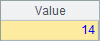A 1 =connect("demo")

 A 1 =connect("demo") 2 =demo.query("select * from STATES") 3 =A1.query("select * from STATES") 4 >A1.close()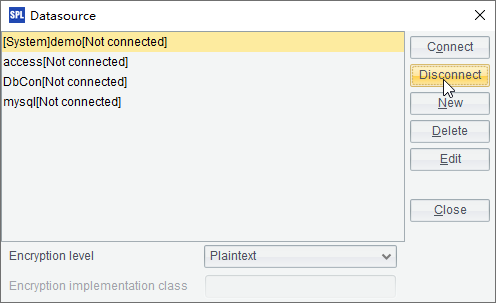## SQL 的简单使用

 A 1 =connect("demo") 2 =A1.query("select * from STATES where ABBR like 'N%' order by POPULATION desc") 3 >A1.close()

A2中查询缩写以N开头的州，并按人口降序排序，结果如下：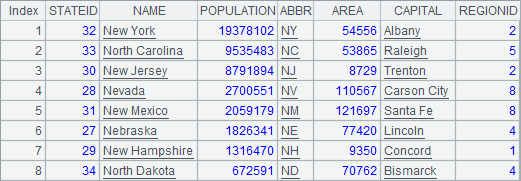SQL语句中，也可以使用网格中的其它数据作为参数：

 A 1 =connect("demo") 2 [CA,ME,NM,SC,LA] 3 =A1.query("select * from STATES where ABBR in (?) order by AREA",A2) 4 >A1.close()

A3中查询缩写在指定序列内的州，并按面积升序排序，结果如下：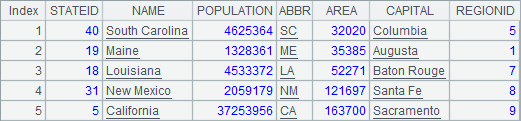A 1 =demo.query@1("select * from CITIES") 2 =demo.query@1("select NAME from CITIES where STATEID=5")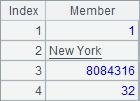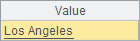## 无返回值的 SQL

 A 1 =connect("demo") 2 >A1.execute("update STATES set ABBR='CAA' where ABBR='CA'") 3 =A1.query("select * from STATES where NAME = 'California'") 4 >A1.close()

A2中的语句修改数据库表STATES中的记录后，A3中查询到的结果如下：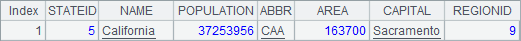A 1 =connect("demo") 2 CA 3 CAA 4 >A1.execute("update STATES set ABBR=? where ABBR=?",A2,A3) 5 =A1.query("select * from STATES where NAME = 'California'") 6 >A1.close()

A4中的语句将数据库表STATES中刚刚被修改的记录改回原值，A5中查询到的结果如下：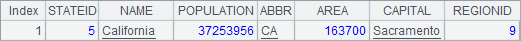## 在单元格中直接使用 SQL

 A 1 \$(demo)select * from STATES where ABBR like 'N%' order by POPULATION desc 2 [CA,ME,NM,SC,LA] 3 \$select * from STATES where ABBR in (?) order by AREA;A2

 A 1 \$(demo) create table TESTTEMP (ID int, NAME varchar(20)) 2 \$(demo) insert into TESTTEMP values (2,'Tom Smith') 3 =demo.query("select * from TESTTEMP") 4 \$(demo)update TESTTEMP set NAME='Jane White' where ID=2 5 =demo.query("select * from TESTTEMP") 6 \$(demo)drop table TESTTEMP

A1中新建一个序表，A2中插入1条记录。A4中修改记录中的数据，A6中删除序表。在A3A5中查询到的数据库更新情况如下：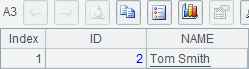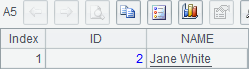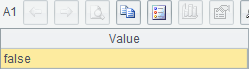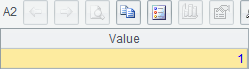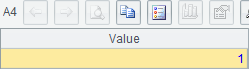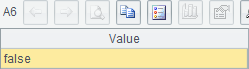## 利用 SQL 的查询结果

 A 1 =demo.query("select * from STATES order by POPULATION desc")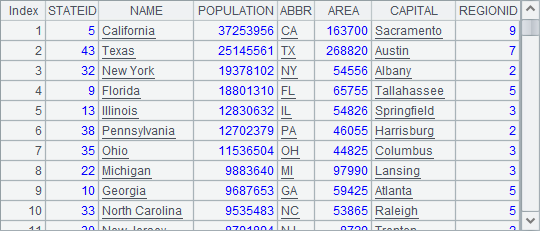A 1 =demo.query("select * from STATES order by POPULATION desc") 2 [CA,ME,NM,SC,LA] 3 =A1.select(A2.pos(ABBR)>0) 4 =A1.select(A2.contain(ABBR))

A3中用A2.pos(ABBR)>0来判断一个州的缩写是否在制定序列中。判断某个数据是否是指定序列的成员，也可以用A.contain(x) 函数，A3A4中的表达式是等价的，运算结果也是相同的，如下：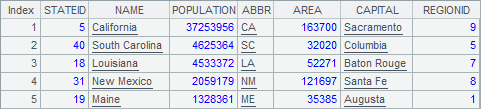A 1 =demo.query("select * from STATES order by POPULATION desc") 2 =A1.count(left(ABBR,1)=="C")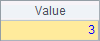A 1 =demo.query("select * from STATES order by POPULATION desc") 2 =A1.group(left(ABBR,1))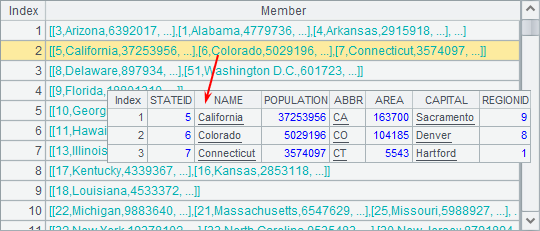A2按照每个州缩写的首字母分组，其中每一组的数据，都可双击查看内容。

 A 1 =demo.query("select * from STATES order by POPULATION desc") 2 =A1.group(left(ABBR,1)) 3 =A2.select(~.count()>=3) 4 =A3.new(left(ABBR,1):Initial, ~.count():Count, ~.sum(POPULATION):TotalPopulation)

A4中的最终结果是：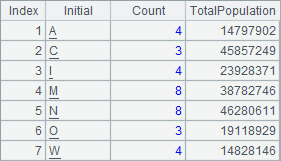## 常见的 SQL 语句与集算器语法的对照

1. select * from

 A 1 =demo.query("select * from EMPLOYEE")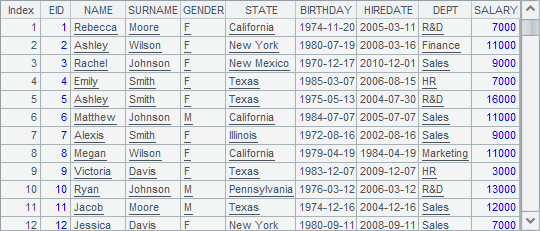2. select … from

 A 1 =demo.query("select * from EMPLOYEE") 2 =A1.new(EID, NAME, SURNAME, GENDER, BIRTHDAY, DEPT) 3 =demo.query("select EID, NAME, SURNAME, GENDER, BIRTHDAY, DEPT from EMPLOYEE")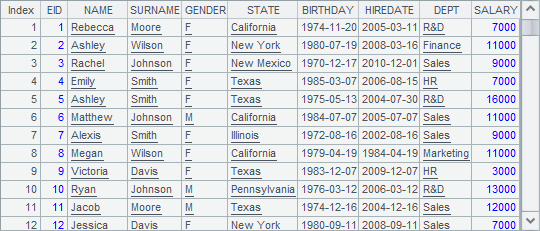3. as

 A 1 =demo.query("select * from EMPLOYEE") 2 =A1.new(EID, NAME+" "+SURNAME: FULLNAME, GENDER, age(BIRTHDAY):AGE, DEPT) 3 =demo.query("select EID, NAME+' '+SURNAME as FULLNAME, GENDER, year(now())-year(BIRTHDAY) as AGE, DEPT from EMPLOYEE")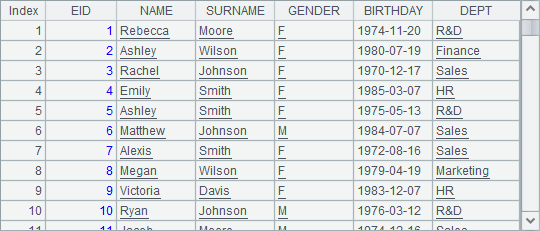4. where

 A 1 =demo.query("select * from EMPLOYEE") 2 =A1.new(EID, NAME+" "+SURNAME: FULLNAME, GENDER, age(BIRTHDAY):AGE, DEPT) 3 =A2.select(AGE<35) 4 =demo.query("select EID, NAME+' '+SURNAME as FULLNAME, GENDER, year(now())-year(BIRTHDAY) as AGE, DEPT from EMPLOYEE where year(now())-year(BIRTHDAY)<30")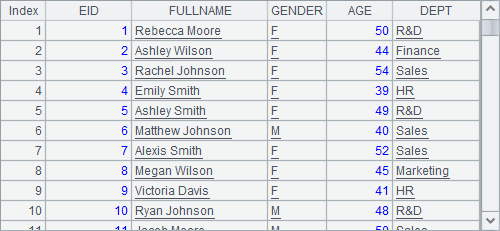A4中用SQL查询同样的结果，语法就复杂得多，而且在这里由于计算年龄时不精确，因此结果也存在误差。

5. countsumavgmaxmin

 A 1 =demo.query("select * from EMPLOYEE") 2 =A1.new(EID, NAME+" "+SURNAME: FULLNAME, GENDER, age(BIRTHDAY):AGE, DEPT) 3 =A2.count(AGE<35) 4 =demo.query("select count(EID) from EMPLOYEE where year(now()) - year(BIRTHDAY)-(case when month(now())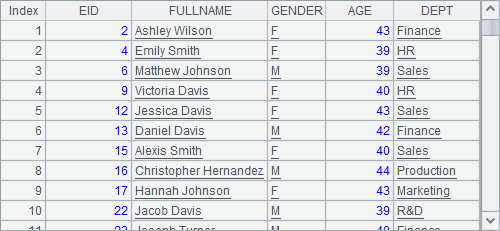A4中这次用比较精确的方法来计算年龄，获得的查询结果和A3中的一致，但无法利用已有的结果，而语句也复杂得多。

sumavgmaxminSQL函数的使用方法和count基本类似。

6. distinct

 A 1 =demo.query("select * from EMPLOYEE") 2 =A1.id(DEPT) 3 =demo.query("select distinct DEPT from EMPLOYEE")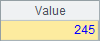7. order by

 A 1 =demo.query("select * from EMPLOYEE") 2 =A1.new(EID, NAME+" "+SURNAME: FULLNAME, GENDER, age(BIRTHDAY):AGE, DEPT) 3 =A2.select(AGE<35).sort(-AGE, FULLNAME ).new(FULLNAME, AGE) 4 =demo.query("select FULLNAME, AGE from (select NAME+' '+SURNAME as FULLNAME, year(now())-year(BIRTHDAY)-(case when month(now()) < month(BIRTHDAY) then 1 WHEN month(now())=month(BIRTHDAY) and day(now())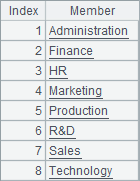8. andornot<>

 A 1 =demo.query("select * from EMPLOYEE") 2 =A1.new(EID, NAME+" "+SURNAME: FULLNAME, GENDER, age(BIRTHDAY):AGE, DEPT) 3 =A2.select(AGE<35&& left(FULLNAME,1)== "S" ).new(FULLNAME, AGE) 4 =demo.query("select FULLNAME, AGE from (select NAME+' '+SURNAME as FULLNAME, year(now())-year(BIRTHDAY)-(case when month(now()) < month(BIRTHDAY) then 1 WHEN month(now())=month(BIRTHDAY) and day(now())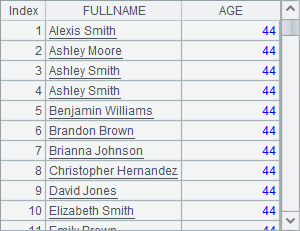9. like

 A 1 =demo.query("select * from EMPLOYEE") 2 =A1.select(like(NAME,"*a")).new(NAME+" "+SURNAME:FULLNAME) 3 =demo.query("select NAME+' '+SURNAME as FULLNAME from EMPLOYEE where NAME like '%a'")10. group

 A 1 =demo.query("select * from EMPLOYEE") 2 =A1.new(NAME+" "+SURNAME: FULLNAME, DEPT).group(DEPT) 3 =A1.groups(DEPT;count(~):ECOUNT) 4 =demo.query("select DEPT, count(*) as ECOUNT from EMPLOYEE group by DEPT order by DEPT")A3中用集算器函数直接计算分组汇总，A4SQL计算分组汇总，它们的结果是相同的。在SQL中，其实并没有真正的“组”的概念，只能在查询中，根据分组直接聚集计算。结果如下：